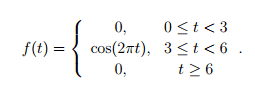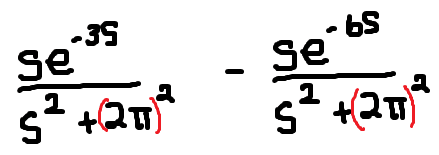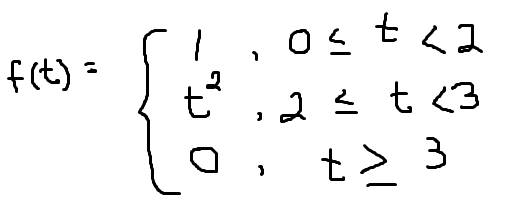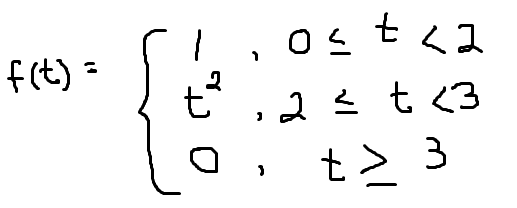# Heaviside and Laplace Transforms

## Homework StatementRearrange f(t) using Heaviside Step Function
Then Rearrange it so that the Laplace Transform can be written down
Then, Write the Laplace Transform of f(t)

## The Attempt at a Solution

So my first step is as follows...
Using the basic Piecewise Function in terms of Unit Functions formulae

f(t) = 0 -cos(2∏t)H(t-3)-cos(2∏t)H(t-6)

Which I've then rearranged into...

f(t) = 0 -cos(2∏(t-3))H(t-3) - cos(2∏(t-6))H(t-6)

Then transformed into...

Sorry, couldn't use the Math editor for this :/
http://img837.imageshack.us/img837/9594/1bff76145aea4f8f8f2dcbd.png [Broken]

Is this right? All help appreciated thanks!

Last edited by a moderator:

BruceW
Homework Helper
I think the second and third terms are correct. But why is the first term there? In the original function,
f(t) = - H(t-3) + cos(2∏(t-3))H(t-3) - cos(2∏(t-6))H(t-6)
The second and third terms make sense to me. But why the first term? Try testing out the function with several possible values of t, to make sure it has the values you want it to have.

HallsofIvy
Homework Helper
If 3< t< 6 then H(t- 3)= 1 and H(t- 6)= 0 so f(t)= -1+ cos(2pi(t- 3)).

That "-1" is not in the original function and I do not understand where the first H(t- 3) came from.

Ah, it's a mistake carried over from a previous homework question I've done.

How would you go about substituting in values of 't' in order for it to validate my f(t) value?

For example...

cos(2∏(t-3))H(t-3) - cos(2∏(t-6))H(t-6)

And substitute in 0,

cos(2∏(0-3))H(0-3) - cos(2∏(0-6))H(0-6) is supposedly = 0
= H(0-3) - H(0-6)
Not sure how you'd solve since there's a stepside function in there.

EDIT:

I didn't follow off my original Piecewise Function to Unit Functions formulae, it's a mistake carried over from a previous question I've done.

BruceW
Homework Helper
H(-6) is just zero, because the input to the H function is less than zero. (that's just from its definition). I'm guessing you know that, but just forgot. It is easy to forget what these 'functions' mean when they are not simple functions like x^2 +4x

Ray Vickson
Homework Helper
Dearly Missed

## Homework StatementRearrange f(t) using Heaviside Step Function
Then Rearrange it so that the Laplace Transform can be written down
Then, Write the Laplace Transform of f(t)

## The Attempt at a Solution

So my first step is as follows...
Using the basic Piecewise Function in terms of Unit Functions formulae

f(t) = 0 -cos(2∏t)H(t-3)-cos(2∏t)H(t-6)

Which I've then rearranged into...

f(t) = 0 -cos(2∏(t-3))H(t-3) - cos(2∏(t-6))H(t-6)

Then transformed into...

Sorry, couldn't use the Math editor for this :/
http://img837.imageshack.us/img837/9594/1bff76145aea4f8f8f2dcbd.png [Broken]

Is this right? All help appreciated thanks!

If ##2 \pi^2## means ##(2 \pi)^2## then yes, your answer is OK; otherwise it is not. If you DO mean ##(2 \pi)^2## you must either use parentheses, or expand it out to get ##4 \pi^2##.

Last edited by a moderator:
Thanks BruceW, unfortunately, I did not know that, going through my lecture notes and slides, there's no information on double checking our Laplace Transforms. Thanks a lot for telling me this, I'll Google it later to do a little bit more research on it!

And Ray Vickson, much appreciated, I missed out this small detail, very obvious, but, didn't catch on!

Muchly appreciated all!BruceW
Homework Helper
yeah, that's OK. happy to help. Also it was good practice for me to check your answer, since I kindof have avoided Laplace transforms in the past. I always felt that the rules were sort of arbitrary and felt like "what is the point of doing all these transforms?"

But now that I know a bit about contour integration, I see some of the rules are not so arbitrary. (like where the 'region of convergence' is). And now that I have experience in doing differential equations, I see that it can be useful to do transforms, so we have an algebraic equation instead of a differential equation.

There is still loads of stuff I don't fully know. Like how there are several possible formal definitions, depending on if you want to talk about Lebesgue integrals or conditionally convergent improper integrals, or in the sense of a distribution. And how the two-sided Laplace transform relates to the Fourier transform - which is probably my favourite transform :)

Anyway, I guess this is all deeper stuff, and most of the time it is fine to just know the 'shallower' stuff. I have come to terms with the fact that I don't know most of the deeper reasons behind it all. As I guess all of us have had to do at some time. (except maybe the 'pure' mathematicians).

Sorry for the massive tangent I have just gone off on.

Aha, that's not a problem at all, I'm assuming a lot of what you've just mentioned is something I'll be coming across in my course :)

This was my first homework question, I assumed it was correct since the tutorer looked at it and ticked me off, but, this is the question where I dragged my error from... Attempt #2(t²-1)H(t-2) + (0-t²)H(t-3)
(t²-1) = (t-2)²+4(t-2)+4-1
H(t-2) [(t-2)²+4(t-2)+3]

and

t²H(t-3) = (t² - 3 + 3)²H(t-3)
[(t-3)²+6(t-3)+9]H(t-3) = H(t-3)(t-3)² + 6H(t-3) + 9H(t-3)
into this
H(t-2)(t-2)² + 4H(t-2)² + 3H(t-2) - H(t-3)(t-3)² + 6H(t-3) + 9H(t-3)

Laplace Transform into this...
http://img24.imageshack.us/img24/9443/2a5314ca4f9c4d748b92907.png [Broken]

Last edited by a moderator:
Ray Vickson
Homework Helper
Dearly Missed
This was my first homework question, I assumed it was correct since the tutorer looked at it and ticked me off, but, this is the question where I dragged my error from... Attempt #2(t²-1)H(t-2) + (0-t²)H(t-3)
(t²-1) = (t-2)²+4(t-2)+4-1
H(t-2) [(t-2)²+4(t-2)+3]

and

t²H(t-3) = (t² - 3 + 3)²H(t-3)
[(t-3)²+6(t-3)+9]H(t-3) = H(t-3)(t-3)² + 6H(t-3) + 9H(t-3)
into this
H(t-2)(t-2)² + 4H(t-2)² + 3H(t-2) - H(t-3)(t-3)² + 6H(t-3) + 9H(t-3)

Laplace Transform into this...
http://img24.imageshack.us/img24/9443/2a5314ca4f9c4d748b92907.png [Broken]

In questions of this type I, personally, find the widespread use of H to be not worth the bother: it often just complicates things and gets in the way. IMHO it is much easier to just use the original definition:
$$\cal{L}(f)(s) = \int_0^{\infty} e^{-st} f(t) \, dt = \int_1^2 e^{-st} 1 \, dt + \int_2^3 e^{-st} t^2 \, dt.$$
However, do whatever seems easiest to you; I just wanted to draw your attention to an alternative.

Last edited by a moderator:
Fair enough then, I have a Chemistry Prac in a bit so I don't have much time to calculate it, but, would the end result come out to as what I have gotten?

Or is there a mistake that needs to be corrected here?

Thanks, I appreciate the feedback.

vela
Staff Emeritus
Homework Helper
This was my first homework question, I assumed it was correct since the tutorer looked at it and ticked me off, but, this is the question where I dragged my error from... Attempt #2(t²-1)H(t-2) + (0-t²)H(t-3)
(t²-1) = (t-2)²+4(t-2)+4-1
H(t-2) [(t-2)²+4(t-2)+3]

and

t²H(t-3) = (t² - 3 + 3)²H(t-3)
[(t-3)²+6(t-3)+9]H(t-3) = H(t-3)(t-3)² + 6H(t-3) + 9H(t-3)
into this
H(t-2)(t-2)² + 4H(t-2)² + 3H(t-2) - H(t-3)(t-3)² + 6H(t-3) + 9H(t-3)

Laplace Transform into this...
http://img24.imageshack.us/img24/9443/2a5314ca4f9c4d748b92907.png [Broken]
You made a few sign errors on the H(t-3) terms because you didn't use parentheses where you were supposed to. Also, you left out the H(t) term.

Last edited by a moderator:
Ray Vickson
Homework Helper
Dearly Missed
Fair enough then, I have a Chemistry Prac in a bit so I don't have much time to calculate it, but, would the end result come out to as what I have gotten?

Or is there a mistake that needs to be corrected here?

Thanks, I appreciate the feedback.

Yes, there are some serious errors. You have
$$\frac{9+3}{s} e^{-3s} + \frac{6+4}{s^2} e^{-2s} +\frac{2}{s^3}e^{-2s} - \frac{2}{s^3} e^{-3s} = \frac{12}{s}e^{-3s} + \frac{10}{s^2} e^{-2s} + \frac{2}{s^3}e^{-2s} - \frac{2}{s^3}e^{-3s}\\ = \left(\frac{10}{s^2} + \frac{2}{s^3}\right) e^{-2s} + \left( \frac{12}{s} - \frac{2}{s^3}\right) e^{-3s} .$$
However, the correct result is
$$\frac{1}{s}e^{-s}+\left( \frac{3}{s}+\frac{2}{s^3}+\frac{4}{s^2}\right) e^{-2s} -\left( \frac{9}{s}+\frac{6}{s^2}+\frac{2}{s^3}\right) e^{-3s}$$